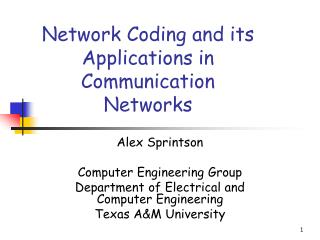DownloadDownload PresentationNetwork Coding and its Applications in Communication Networks

# Network Coding and its Applications in Communication Networks

Télécharger la présentation## Network Coding and its Applications in Communication Networks

- - - - - - - - - - - - - - - - - - - - - - - - - - - E N D - - - - - - - - - - - - - - - - - - - - - - - - - - -
##### Presentation Transcript

1. Network Coding and its Applications in Communication Networks Alex Sprintson Computer Engineering Group Department of Electrical and Computer Engineering Texas A&M University

2. NP-hardness result • Lemma (Rasala et. al. 2003). Deciding whether there exists a linear network code with alphabet size q for a multicast network coding instance is NP-hard when q is a prime power.

3. NP-hardness result • Proof: Reduction from the chromatic number problem s y S1 x+y S1 y x y s1 s2 s3 s4 x+0*y S2 S2 S3 S3 x+1*y S4 T4 T5 S4 T1 T2 T3 qmin= least prime power > X(G)-1

4. Cyclic networks • In certain settings, cycles are necessary

5. Quiz • Given a network (G,s,T) with minimum cut h, is it possible to transmit h packets to all T terminals if each link can only transmit one packet? • Always yes, if the network is acyclic

6. Quiz • Sometimes, if the network has cycles

7. Quiz • These networks are not really cyclic:

8. Quiz • A example of a “truly” cyclic network • We prove: it is impossible to send two packets in one round to all destinations Proof: By way of contradiction

9. Quiz • Let e be the first link of the cycle v1->v2->v3->v4->v1 that transmits a packet. • Case study. If e=(v1,v2) then t1 gets no information about b

10. Solution • We have shown that it is not possible to send two packets in one round to both terminals • However, it is possible to send 2n packets in n+1 rounds • Asymptotically, the rate is two packets per round • Use convolution codes

11. Convolution Codes • Idea: mix messages from different rounds

12. Convolution Codes • Recovery at terminal t1

13. Algebraic framework Ax=z aij,xi,ziGF(2n) Observation: Any two rows of matrix A must be linearly independent

14. Algebraic framework (cont.) A4x=z Observation: Matrices A1, A2,…,Akmust be of full rank (invertible)

15. Notation Y(e) All operations in the network are linear

16. Intermediate source At the intermediate nodes All operations in the network are linear

18. Example 1

19. Example 2

20. Transfer matrix

21. Transfer matrix • Observation: The information propagates throughout networks on paths with different length. • Length of the path - the number of hops between the source and the tail of the edge. • For example, for edge e5 there are two paths - one through path (e2,e5) and one through path (e1,e3,e5)

22. Transfer matrix • Let be the vector of packets transmitted over all links • Let be the contribution (gain) of paths on length i: • Note that

23. Transfer matrix • Contribution of paths of length zero:

24. Contribution of paths of length one

25. Contribution of paths of length two

26. Transfer matrix

27. Transfer matrix

28. Transfer matrix

29. Transfer matrix

30. Transfer matrix

31. Transfer matrix

32. Linear network system

33. Example 3 Point-to-pointconnections

34. Example 3

35. Point-to-Point connections Define matrices A, B, and M

36. Point-to-Point connections Idea: Choose parameters in an extension field such that the determinant of is nonzero over Then, we can set A to be the identity matrix and B so that M is the identity matrix

37. Critical property The equation Admits a choice of variables over so that the polynomial does not evaluate to zero!!

38. Point-to-Point connections A possible solution: Set And set to be equal zero. Equivalent to finding three disjoint paths between s and t!

39. An algebraic max flow min cut condition [KM01, 02, 03]

40. Recap (from Wikipedia) ( )

41. Polynomial Ring Let F be a field. The set of all polynomials with coefficients in the field F, together with the addition and the multiplication forms itself a ring, the polynomial ring over F, which is denoted by F[X].